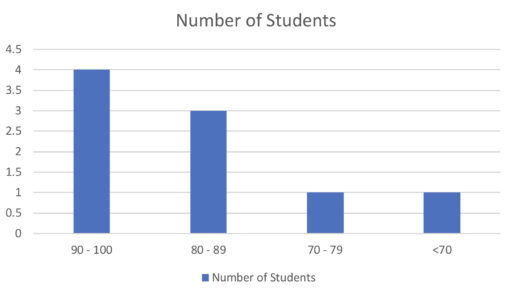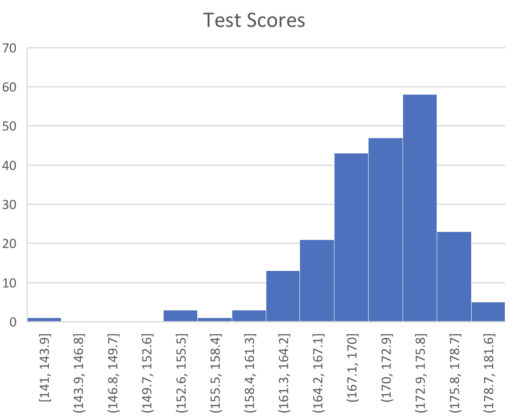A histogram looks a lot like a bar graph and, indeed, you can think of it as a special kind of bar graph. But instead of having just any kind of category (as a bar graph does), histograms have, as their categories, certain ranges of values. So, for example, suppose the students in your class score the following scores on their test:Now, those numbers are kind of unwieldy. So to get a sense of how many students are acing your class (getting an A) or failing your class (getting an F), you might categorize their test scores according to certain ranges. So put all the 90-100 scores together in one category, all the 80 - 89 scores in the same category, and so on. Graphing this, we would get the following:This graph tells us that 4 students scored between 90 and 100; 3 students between 80 and 89; and so on.

Example 1

In the following histogram, approximately how many students scored a 170 or higher?Example 2

In the below histogram, what if anything can we conclude about the median of the data?Example 3

In the following chart, what can we conclude about the range of the data?Practice Problems

1. In the following histogram, approximately how many students scored a 170 or lower?2. In the below histogram, what if anything can we conclude about the range of the data?3. In the below histogram, what is the minimum possible number of students who scored higher than 75? What about the maximum?